## Example Questions

### Example Question #91 : Coordinate Geometry

Which of the following equations represents a line perpendicular to 3x + 4y = 5?

y = 4x - 2

y = 3x + 2

y = (-4/3)x + 2

y = (4/3)x - 15

y = (3/4)x - 8

y = (4/3)x - 15

Explanation:

Perpendicular lines have opposite and reciprocal slopes.  Therefore, let us find the slope of our line and appropriately modify it to find the perpendicular line.  To find the slope of our line, remember that in the slope-intercept form (y = mx + b), m represents the slope.

3x + 4y = 5  => 4y = 5 - 3x  => y = (-3/4)x + 5/4

Therefore, the slope of our line is -3/4.  The perpendicular to this would be 4 / 3.  Therefore, only y = (4/3)x - 15 is perpendicular.

### Example Question #2 : How To Find The Equation Of A Perpendicular Line

What is the equation for the line passing through the point (5,4) and is perpendicular to the line 8y + 4x = 10?

y = -0.5x – 6

y = -2x + 15

y = 2x – 6

y = 3x + 44

y = x – 6

y = 2x – 6

Explanation:

To solve this, we need to use the point-slope form.  However, to do this, we need to ascertain the slope of the perpendicular line.  For this, get 8y + 4x = 10 into slope-intercept form:

8y = -4x + 10; y = (-1/2)x + 1.25

Therefore, the slope of this line is -1/2 and its perpendicular (opposite and reciprocal) slope is 2.

The point slope form is: (y – y1) = m * (x – x1)

For our data it is: y – 4 = 2 * (x – 5)

Simplifying, we get: y – 4 = 2 x – 10; y = 2x – 6

### Example Question #1 : How To Find The Equation Of A Perpendicular Line

Which of the following is an equation of a line that is perpendicular to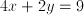?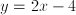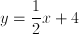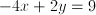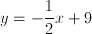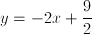Explanation:

Rewrite the equation in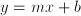form.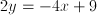The perpendicular line will have a slope which is the negative reciprocal of the slope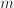.

### Example Question #4 : How To Find The Equation Of A Perpendicular Line

Which of the following is a line perpendicular to the line passing through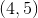and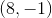?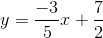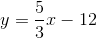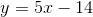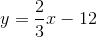Explanation:

To find if something is perpendicular, you need to first know the slope of your given line.  Based on your points, this is easy.  Recall that slope is merely: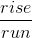This is: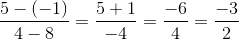Since a perpendicular line has a slope that is both opposite in sign and reciprocal, you need to choose a line with a slope of.  The only possible option is, therefore,### Example Question #5 : How To Find The Equation Of A Perpendicular Line

Which of the following is a line perpendicular to the line passing through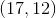and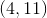?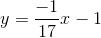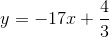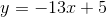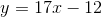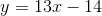Explanation:

To find if something is perpendicular, you need to first know the slope of your given line. Based on your points, this is easy. Recall that slope is merely:This is: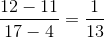Since a perpendicular line has a slope that is both opposite in sign and reciprocal, you need to choose a line with a slope of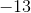.  The only possible option is, therefore,